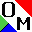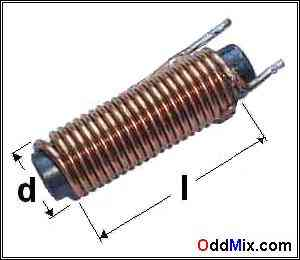OddMix  CIRCUITS - CRYSTAL RADIO

OddMix.com - Technical Note - Crystal Radio - TN080821 - Karl NagyFigure 1. Crystal Radio Coil Design Detail

For most of us willing to try to build a crystal detector radio, the most difficult part of the construction usually is to sort out the coil parameters. In almost all otherwise well written construction articles the coil details are the most hazy and difficult to figure out. Out of the three fundamental building blocks in electronics, inductors are the most complicated and difficult to understand. This small note hopefully helps to determine the nature of the inductors or coils, our most important and difficult components.

Figure 1 shows a single layer simple coil known as a solenoid. In our picture it is shown with a ferrite core. Many of us have seen the formula of self-inductance in physics. Here is a refresher of the long forgotten formula. All length units are in meters. The coil's self inductance L in [Henry];The inductance of a coil is directly proportional to the permeability of free space and/or the permeability of the actual core material used, the square of the number of turns and the area of the turns. The coil inductance is inversely proportional to the length of the coil. Briefly it means that the shorter is the coil, or the smaller of it's diameter the less inductance it has. It also means, that the more turn it has, or if it uses a core that has a higher permeability than air, the higher its inductance would be. Because the l flux length is difficult to measure for all but solenoid and toroid coils, various compensation factors are in use.

```                          L = Self inductance [Henry]
u0= Permeability of free space = 4 Pi x 10-7 [Henry/meter]
ur= Permeability of air = 1 [Henry/meter]
N = Number of turns in the coil
A = Area of the turns [meter squared]
l = Length the flux lines [meter]```

The quality of the coil is the ratio of its reactance XL (the inductive resistance) and its resistance R.

The tuned circuit in a crystal detector radio with a high Q (quality) coil has a narrower pass-band, and that makes it better to pick out a station of many equally strong. A lower Q coil in a tuned circuit has a much wider pass band. It allows more than one station through and makes listening to either radio stations frustrating. Higher Q can be made with a low resistance coil, where the copper wire is thick and as short as possible.

The tuned circuit's coil resistance can be also reduced with an added ferrite core inside it. Thus the increase in inductance allows less copper wire in the coil with less resistance and a higher Q. When a tuned circuit is in resonance the higher the Q, the available RF voltage or the input voltage multiplication number is also higher.Copyright © 2015 K Nagy - http://www.OddMix.com - All Rights Reserved Page Revised: 2015-01-01 - - Privacy Policy - Site Map - Support